Do you like the article?
Share it with others -
Use new possibilities of MetaTrader 5

# Price series discretization, random component and noise

23 November 2020, 07:47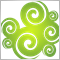0
4 613

### Introduction

The classical method of representing price series as time intervals (or time frames) appeared long ago, at the beginning of formation of financial markets, when there were no computers and when real goods were traded in real markets. Storing every price change during a day was difficult. Moreover, it was useless because prices were not changing quickly. Therefore, the obvious solution was to register price values at regular time intervals. Sounds logical: "Today wheat costs 90 cents, while yesterday it cost 80 cents." Everything is very clear: demand has grown and the price has risen. There were not many deals, as compared to today's market trading, that is why price was redefined rarely.

With the emergence and development of price data analysis, the aim of which was to better predict price behavior, and with an increase in the number of trading operations, it became important for people to understand which was the highest and the lowest price for a certain time period. That is, information about the yesterday's price of 80 cents and of today's price of 90 cents was no longer enough. People wanted to know which highest and lowest prices were reached within the specified period of time. That is when the well-known candlesticks and bars were invented.

As the number of trading operations increased, the price series discretization was becoming more and more accurate. Now, we already use the minute discretization or sometimes even smaller frames, such as a second and ten seconds.

The main advantages of the time discretization of a price series are as follows:

• Convenience. We know exactly that the next bar will be formed in a minute and we will receive new Open, Close, High and Low price values.
• Resource efficiency. The candlestick representation allows storing only 4 digits over a period of time, if greater precision is not required. If you store each change in the bid, ask and last price, the volume of a one-year history would be very large, up to several gigabytes. This would be a real problem if you need to download and store price history for 10-20 years, of if you use not one, but 200-500 symbols. Furthermore, huge computer resources would be needed to process gigabytes of history. This is why candlestick analysis and processing look more attractive.
• Easy scaling and visual analysis. When it is necessary to look at a bigger picture, the discretization scale can be increased to weeks or even months, and the display will show data for as many years as needed. If more precision is required, you can decrease the scale to view what was happening within a minute.
• Linearity in time. Perhaps the most convenient thing in this presentation is that each one-year history interval visually takes about the same screen space. Finding on a chart the previous year or hour is easy and intuitive. Intuitively, linearity in time seems to be a very important parameter, but there are times when the "right" decisions are counterintuitive.
• Ease of price comparison for different instruments.

### Signal Discretization Features

Data discretization is needed not only in trading but also in many other signal processing areas. For example, in music the originally continuous signal is digitized. It is encoded using time discretization. The signal amplitude value at regular time intervals is written to memory. This signal can then be converted back to a continuous signal using certain manipulations. Continuous signal discretization is a well-studied sphere. For example, the rule that follows from the Kotelnikov (Nyquist-Shannon) theorem states: "A signal can be completely restored if the discretization frequency is 2 or more times the signal frequency." So, if a signal frequency is 1 hertz, then its amplitude value must be read at least 2 times per second (that is, with a frequency of 2 hertz). Only in this case it will be possible to obtain its original form after discretization. Figure 1 shows what happens if we discretize a 1 hertz sine wave with a 2 hertz sample rate. The signal is shown in green, and the result of discretization is shown in red.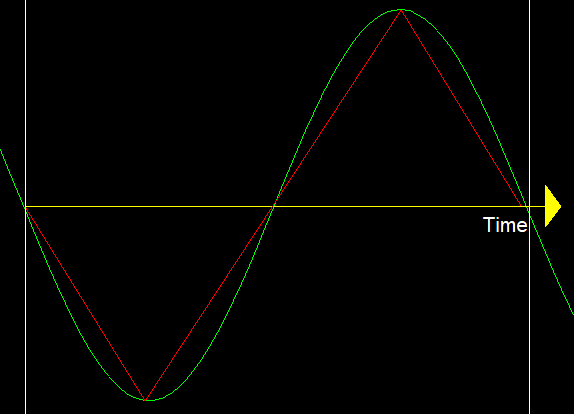Fig. 1.

After discretization, the sinusoid is converted to a triangular waveform. There is some error of course, but this triangular signal can be converted back to a sinusoid using low-pass filters. It means that we can restore a signal, preserving its idea, period and amplitude, though with some error. Such distortions are considered critical in music, but they are not so relevant for trading. But what if the discretization rate is less than the original signal rate? An example is shown in figure 2 below.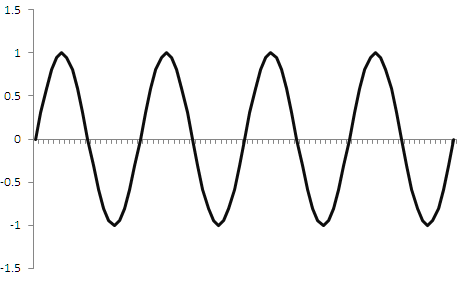---->>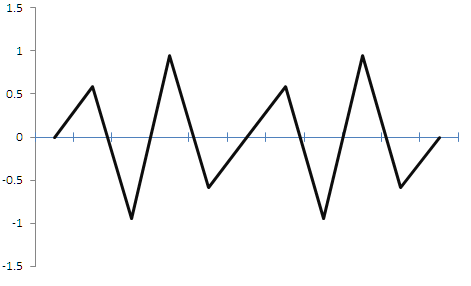Fig. 2.

The figure shows that if the discretization frequency is less than a double signal frequency, the resulting signal is greatly distorted, and we actually receive a random signal which has nothing to do with the original signal. When applied in trading, the first case would allow us to sell when we find High and to buy when we see Low. Also, in this case we know the frequency. After the incorrect discretization, we lose information about the amplitude and the signal frequency. A deterministic periodic signal with known characteristics turned into a random non-periodic signal with unknown characteristics due to an incorrectly chosen discretization rate.

Two logical questions arise from the above knowledge: "Aren't we making a mistake when discretizing a price series?" and "Is the price series a discrete or a continuous signal and what are its parameters?"

The answer is not that simple, and it is very important.

### Is the Price Series Discrete or Continuous?

The question can be answered if we know the market price forming mechanism. I will not describe it in detail, as the description is provided in the article "Principles of Exchange Pricing through the Example of Moscow Exchange's Derivatives Market". Some participants place orders in the Market Depth, and other participants buy the required amount at the required price. This is what happens when a price chart is formed. The levels are discrete, i.e. it is possible to place an order at a price of 1, 2, 3 and so one, with certain accuracy. The volume set in bids and purchased by buyers is also discrete, because you can buy 1, 2, 3 or more units. Figure 3 below shows an example of the Depth of Market; you can see that prices and volumes have discrete values.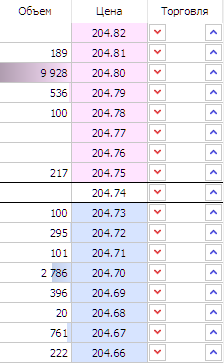Figure 3.

Hence, we can conclude that the price series chart is discrete in nature. The price moves along discrete levels after participants buy discrete volume amounts.

### The Function of What Is the Price

We found out that the price series itself is discrete, but the function of which parameter is the price change?

Discretization of an audio signal at regular intervals is an acceptable solution because an audio signal is a function that changes over time. The signal itself is an amplitude which depends on time. This signal property is fundamental. That is why there are no problems here.

The price series has a different nature. Here, the amplitude (the price) changes over time, but the time is not the reason for the price change. If you try to figure out the reason for the price change, then the question is not that simple. Several assumptions can be made:

1. Price as a function of trades. The price changes in accordance with performed trades because trading operations move the price. But trading operations may not lead to price changes. For example, 10 stocks are available at a price of USD 1. A participant purchases 4 stocks, and there are 6 stocks left at the same price. So, a trading operation was executed, but the price has not changed. However, the operation has decreased the volume available at this price, which can lead to further price change, when the next participant buys the entire volume. Prices only change when the amount of available stocks at USD 1 is not enough to cover the demand and the entire available amount was bought out. In this case, the ASK price moves to USD 1.1. However, other participants are still able to place an order at USD 1 and move the ASK price back.
2. Price as a function of all trading operations in the market. The price can change not only when participants buy out the volume at a certain level, but also when they simply cancel their orders or move them to other levels. Thus, there will be no trading operations, but the BID and ASK prices will move.
3. Price as a function of "benefit". The price can change because the participants redefine the asset value for themselves. There can be absolutely different reasons for redefining the asset value. In any case, the redefinition of the asset value is closely related to the benefit of the participants. In theory, the established price is the most beneficial one for the entire set of participants, including buyers and sellers (even if this benefit is negative). The market was originally created to maximize the benefits and to determine the optimal equilibrium price which is acceptable both for the buyer and the seller. This benefit may not be related to a certain asset. For example, an investment fund needs to urgently sell an asset. They are ready to do this even if they will have losses, because they may receive some other benefit by executing this operation, such as they will be able to buy another asset or to pay out profits to their clients. The nature of the benefit can be different. In such a case, the price is a function of each redefinition of the benefits for each participant.
4. Price as a function of itself. Obviously, every price change leads to a change in the benefits for the market participants. Benefits can change without changing the price, but when the price changes, the benefits of the participants change. This is not the most accurate description of price changes, but it allows us to roughly approach an ideal model and to make some assumptions which will not have a great effect on results in future. We basically trade price changes. Even if we select dividend strategies (ignoring the price), payment of dividends will ultimately lead to price changes. In this case, the price change is a signal to shift the chart to the left. Register price changes only when the price moves one point. Any step can be used depending on the preferred scale: price values can be registered every time when it moves n points up or down.

I consider the third option most probable, stating that price is a function of redefining benefits. But it is impossible to calculate the benefit of each participant in order to discretize the series. In the first two cases, it is possible to calculate trading and non-trading operations in exchange markets, but there can also be difficulties. For example, an asset can be traded in two or more different exchanges. Or if derivatives of an asset exist, such as futures and options, do we need to calculate the operations which are indirectly connected with the asset? These questions require a separate large study. In any case, all the four cases are indirectly related to each other. The fourth option, where price is a function of itself, allows further research, provided that we assume this to be a rough model.

The rate of change in the asset price depends on the number of trades. The more trading operations, the more frequently the price changes, which means there is a direct correlation. Accordingly, if there are a lot of participants in the market, they make a lot of trading operations which leads to a more frequent redefinition of benefit for each participant. So, every participant will try to redefine the price more frequently, leading to a larger number of trades, and the frequency of the asset price change will be high.

### Features of Price Series Discretization by Time Intervals and the Random Component

According to our rough model, the price is a function of itself, and we discretize the price only when it changes by a certain number of points. Even if this is not quite true, this assumption will enable further understanding of the topic without affecting the final result. Furthermore, in order to profit, we need to know that the price has changed. Additionally, we need to know how it has changed in order to search for patters.

Each 1-point price change (here a point is the smallest possible price change) will be equal to one step. Let us see what happens when such a series is time discretized. Obviously, the number of points that the price will pass per unit of time depends on the trading activity. The higher the trading activity (the number of trading operations performed), the more steps the price will eventually take. Trading activity is not directly related to price movement, but price movement depends on trading activity: the higher the activity, the more price movements there will be. The dependence is indirect, but the correlation is positive. Suppose 1 step is 10 points. One-hour candlesticks will be used in the example. The two figures below show prices represented as bocks. The blocks are similar to Renko bars, but they are constructed according to a slightly different principle. They use High and Low of classic candlesticks, which show the highest and lowest price values for the block formation time. Like candlesticks, blocks have 4 characteristics: Open, High, Low and Close. The difference from candlesticks is that the distance between Open and Close is always fixed and is expressed in points. The block closes when the price passes N points up vertically. For example, the block size is 10 points. Once the price moves 10 points vertically, the block closes and a new block begins.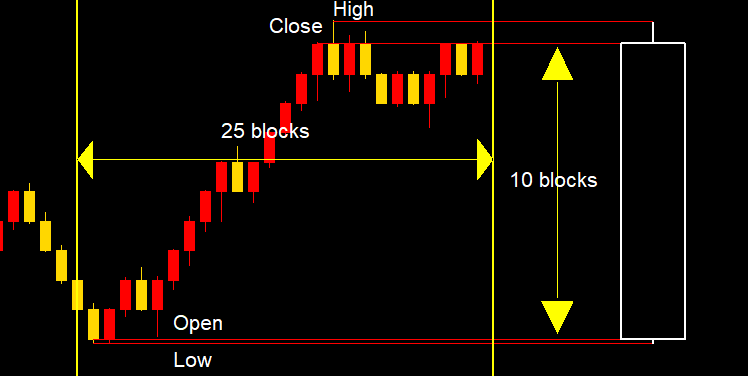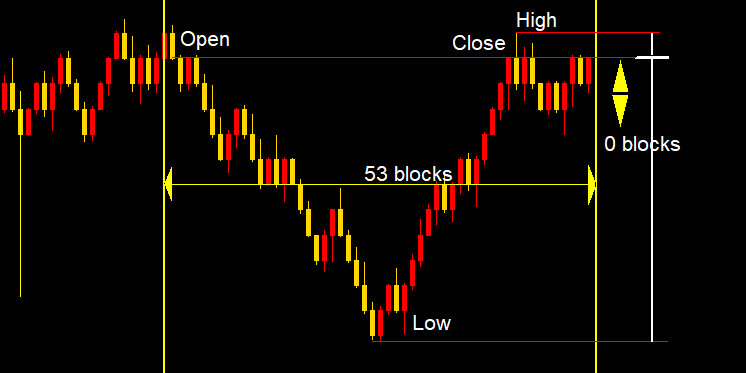Figure 4.

If one block is equal to one step of N points, then let us see what happens inside a one-hour candlestick. Figures 4 show the movements which happened during the formation of the one-hour candlestick. In the upper figure, the price passed 10 blocks vertically in one hour. If block size is 10 points, then the candlestick size is 100 points. Totally, 25 blocks were formed for the whole candlestick formation time, which is equal to 25 steps. Another example is shown in the lower figure, in which the price moved 0 blocks or 0 points during the candlestick formation time. Totally, the price moved 40 blocks, or 40 steps, during the price formation time. Now, let us view the chart that consists of such candlesticks. It is shown in figure 5. The candlestick closes once an hour, and the price series is definitely not a function of time. In a simplified case it is a function of trading operations, which have a positive correlation with the number of points passed. Since we converted the points into blocks, the number of blocks has a positive correlation with the number of trades. Actually, each candlestick (suppose one hour) turns out to have a random number of blocks, or steps N point each. Further we will see why this is important. This is because candlestick simply close after certain time, despite the processes taking place in the market. Time is an external parameter which has little relation to price related processes. In other words, the price changes not because an hour has passed, but for some other reasons, one of which can also be the elapsed time.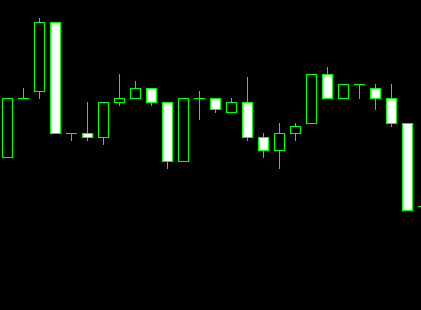Figure 5.

Trading activity can change significantly over time. Furthermore, trading activity of different trading instruments is be different. In addition, the development of algo-trading leads to an increase in the number of operations per time unit and in trading turnover, which means that the direct comparison of candlesticks between year 2010 and 2020 would be incorrect, because each of them would contain a different number of operations.

Now, let us make one more simplification and take the market for a random walk. Of course, the market is not a random walk, but this way it will be easier to understand. Later, we will get back to real markets.

The Central Limit Theorem states that the sum of a sufficiently large number of weakly dependent random variables having approximately the same scales (none of the terms dominates or makes a determining contribution to the sum) is approximately normally distributed. As applied to our case, we can conclude from this theorem that, on average, our random process will pass for N steps a distance which is vertically proportional approximately to the square root of the number of steps. If one block is 1 step, then for 100 blocks the price will pass vertically 100^0.5 = 10 blocks, on average. It can be more or less in isolated cases, but on average, the random series will follow the normal distribution rule. The number of steps of this random series will slightly depend on time, because the steps are generated by the price activity, which can vary greatly over time.

Thus, the size of the one-hour candlestick for such a random price series will, on average, be proportional to N^0.5, where N is the number of steps inside the candlestick. It means that one-hour candlestick size will be subject to the normal distribution law. Further, taking into account the fact that a random number of steps can exist within a candlestick, we can conclude that the candlestick size will also be subject to the normal distribution law. I.e. the candlestick size is equal to a square root of the number of steps within this candlestick. Let us check this statement. For this purpose, I will use 50,000 1-minute GBPUSD candlesticks for a period between 2020.05.18 and 2020.07.03.

1. Let us find the modulo value of the average size of a 1-minute candlestick - this will be the step size. To do this, subtract the closing price of each previous candlestick from the closing price of each next candlestick and take the modulo value. I got the average size of a 1-minute GBPUSD candlestick = 0.000170
2. Now, let us find the average size of a one-hour candlestick for the same period (a one-hour candlestick contains 60 1-minute candlesticks). To do this, subtract the closing price of each previous one-hour candlestick from the closing price of each next one-hour candlestick and take the modulo value. I got the average size of a one-hour GBPUSD candlestick = 0.001117
3. Let us find now how much the price should pass per hour on average, if we assume that the price series is a random walk. To find this, multiply the average size of a 1-minute candlestick by a square root of the number of steps, and multiply by the average step size. We have 60 => 0.000170*(60^0.5) = 0.001315. The size of a one-hour candlestick would tend to this average size if the original series followed the normal distribution law.
4. Let us compare the average size of a real candlestick and a random walk candlestick (real 0.001117) ≈ (theoretical 0.001315). The difference is only 0.0002. It can be concluded that our assumption that the candlestick size is subject to the normal distribution law true and is confirmed by the real symbol market data. The difference of 0.0002 is not significant.

Further, using the obtained one-hour candlesticks, we actually combine and analyze a sequence of certain segments of a price series, the amplitude of which follows normal distribution law. Naturally, if we move to higher timeframes, we get all the same candlesticks, the size of which is proportional to the square root of the number of steps. Now get back to figure 2: if a series is incorrectly discretized, a random sequence is output as a result. In fact, by using time discretization, we convert a price series into a random sequence. Well, a time discretized sequence can still have patterns, because the market is not a random sequence and it has indirect dependences on time but analyzing such a series and identifying patterns becomes much more difficult. This is the time discretization that leads to the so-called "noises" and non-stationarity in the price series - they are often discussed, but no one explains where those noises come from. Now, noises are not only discretization noises, which appear when a signal is discretized, but they also include a random component which appears because of incorrect discretization parameters of a price series which itself is discrete.

To make sure that the results we obtained with one-hour candlesticks were not an accidental coincidence, let is repeat the same process for daily candlesticks. There are 24 hours a day. Since currency pairs are traded around the clock, let us assume that the daily candlestick contains 1440 1-minute candlesticks. Let us sample the same interval of 1-minute data that we used for H1. This time, the relevant data is represented in a tabular form for convenience.

Average size of M1 candlestick
Average size of H1 candlestick
Theoretical size of H1 candlestick calculated as
0.000117*(60^0.5)
Average real size of D1 candlestick
Theoretical size of D1 candlestick calculated as
0.00017*(1440^0.5)
0.000170
0.001117
0.001315
0.006547
0.006442

As you can see, the real average size of a daily candlestick would not differ much from the theoretically predicted size if it were not a real market, but a random walk. The Excel file with historical data and calculations is attached below.

To calculate the average size of a higher timeframe candlestick, we used 1-minute timeframe data. But what if we get lower to tick data - will this produce a different result? What we are going to do:

1. Take tick volume data of 1-minute candlesticks (from a real account) for the same period and calculate the average number of ticks in a one-minute candlestick - the average number is 59.99 ticks per minute.
2. Load the tick data and find out the average tick size, it is equal to 0.000014378.
3. Calculate the theoretical size of a 1-minute candlestick as (59.99^0.5)*0.00014378=0.000111363
4. Calculate the theoretical size of a one-hour candlestick as ((59.99*60)^0.5)* 0.000014378= 0.00086
Average tick size Average real M1 candlestick size Theoretical size of M1 candlestick calculated as (59.99^0.5)*0.000014378 Average size of H1 candlestick
Theoretical size of H1 candlestick calculated ((59.99*60)^0.5)*0.000014378
0.000014378 0.00017 0.000111363 0.001117 0.00086

As you can see, the average size of the M1 and H1 candlestick size does not differ much from the theoretical one, which the price series would have if it was a random walk. Thus, we can conclude that for some reason the price series behaves like a random walk which can probably be due to an incorrect discretization of time intervals.

### Density of Distribution of Price Series Increments

The quantitative assessment allowed to roughly estimate how the price series candlestick size corresponds to the random walk candlestick size. It is more interesting to plot the density of distributions of price series increments, which will allow us to visually assess the similarities or differences with the density of distribution of random walk increments.

An important part of the analysis is data preparation. The increments density will be calculated for the number of points which the price can move in 60 minutes (in 1 hour), i.e. in 60 steps. Here we need to know the average size of a 1-minute candlestick and the number of steps (the number of 1-minute candlesticks in a one-minute candlestick). We have already calculated the average size of a 1-minute candlestick - it is equal to 0.000170. The number of 1-minute candlesticks in a 1-minute candlestick is 60. It means that the price can make 60 steps per hour, each step being 0.000170. Thus, the extreme cases will be when the price moves continuously 60 points up or 60 points down from the starting point. So, the distribution density will be estimated in the range between -0.00017*60=-0.0102 and 0.00017*60=0.0102. In other words, we know that if the price only moves up, in steps of 0.00017, then it will reach a maximum of 0.0102 in 60 steps (in one hour). Moreover, for 60 steps, the price can only take discrete levels 0, 0.00034, 0.00068, 0.00102 ... 0.0102 and similar negative values. Figure 6 show why the price will take exactly these levels.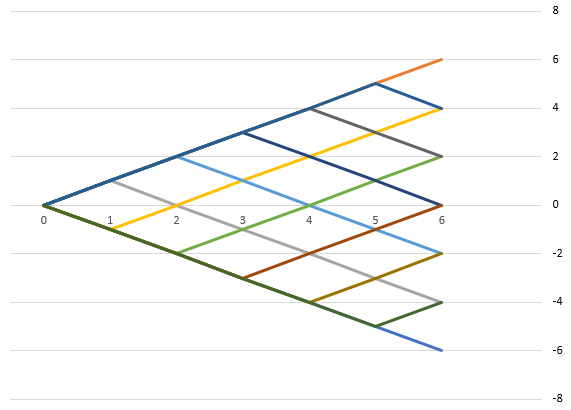Figure 6.

If some discrete function starts at a zero point, its step is equal to 1 and it makes 6 steps, then it can ultimately move vertically 6, 4, 2 or 0 segments and can only take discrete values with a double step size. Our case is similar: if the average size of a 1-minute candlestick is 0.00017, then the price can take discrete levels with an interval equal to twice the size of the candlestick. In reality, candlesticks can have different sizes, not only 0.00017, that is why the price can take intermediate values. Therefore, for analysis we will count how many events fall within the intervals with a double average candle size. In order to find the difference of the resulting distribution for the price series, compare it with the distribution for a random walk. The characteristic of a random walk is that the direction change probability at each next step is 50% and that there is no memory. Figure 7 shows probability density distributions in black for a price series and in red for a random walk.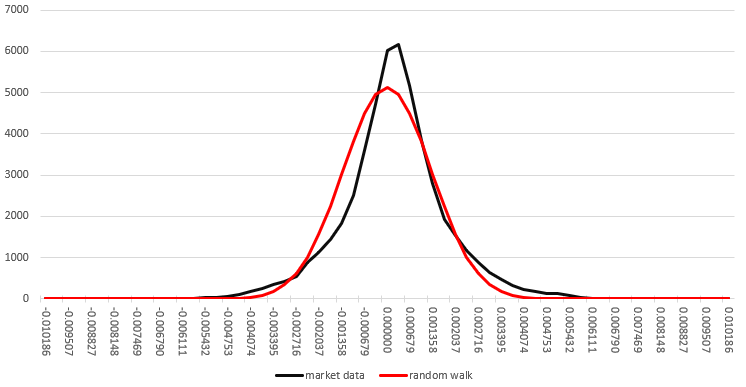Figure 7.

It can be seen from figure 7 that the density of the price series probability distribution almost matches the density of the random walk probability distribution. The density of the price series probability distribution has a slightly higher peak, is slightly narrower and is slightly shifted to the right, as compared to the random walk distribution density. This suggests that the probability of a reversal at each next step in the price series is slightly higher than 50%, and there is a small uptrend, but these differences are not large. It might seem that profiting from this deviation is not so difficult, but we will consider this in the next article. Here we deal with an ideal random variable, which, with a large enough sample, will tend to a benchmark. The graph of the probability density distribution for a random walk is plotted using a table, part of which is shown in Figure 8.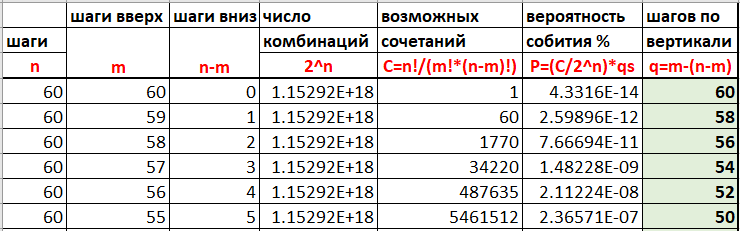Figure 8.

This probability density distribution graph confirms our earlier conclusion that the candlestick size (in this case H1 candlestick) tends to the candlestick size which would be if the price series were a random walk. It also allows assessing this fact visually and more accurately.

All data and calculations mentioned in this article are attached in the xlsx file below.

### High and Low Volatility Areas

Attentive traders may notice that the candlesticks in the market are conventionally divided into groups of "large" and "small" sized candlesticks (areas with high and low volatility), which means that the chart is not a random walk and there are patterns. If time discretization introduced strong distortions, then this effect would not be observed. However, this feature can be explained by the fact that the candlestick size depends on the number of trading operations executed inside this candlestick. How this can be checked? You can simply look at the chart with tick volumes - "small candlestick" periods are accompanied by low tick volumes, and "large candlestick" periods come alongside high tick volume periods. If we assume that one tick is one step, then the price makes more steps during "large candlestick" periods, but the candlestick size remains proportional to the square root of the number of steps multiplied by the average step size (example on Figure 9). Tick volumes, in turn, are directly correlated with the trading activity: the higher the trading activity, the more ticks pass per unit of time. Exchange markets provide the history of real volumes, where you can see that tick volumes strongly correlate with real volumes, which also indicates the relationship between the number of ticks and the intensity of trading activity.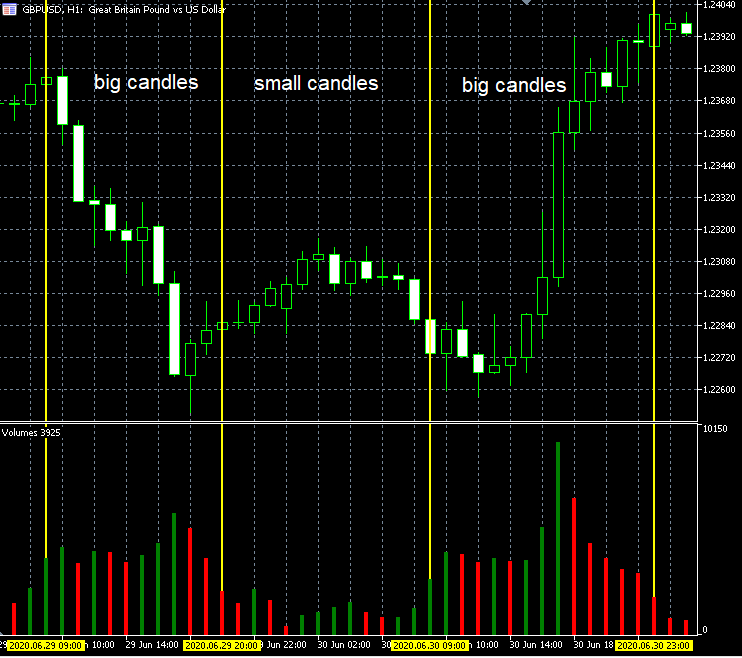Figure 9.

This also proves that time discretization introduces strong distortions to the resulting price series form and thus it makes price analysis more difficult. This distortion has led to the emergence of a separate category of trading systems, the so-called "night scalpers". I am not saying that they cannot generate profits, but it is necessary to take into account this peculiarity of a price series presentation when developing such systems and to conduct additional research in order to answer the following question: "Is the probability of a reversal in these areas really higher than the probability of a trend continuation?" and "Do the statistical characteristics of these areas allow to generate a profit?", because such systems are based on this pattern.

### Alternative Price Series Discretization

The simple conclusion of the above analysis suggests that tick data is more suitable for processing and analysis, as they avoid discretization errors in a price series. If we need a larger scale, we will use blocks of 10 or 100 ticks. But the problem is that ticks themselves are also a method of discretization. This method is widely used, but it still can introduce distortions in the process because the price is not a function of received ticks. The price is at least a function of executed trades, while a trade may not always generate a tick. Ticks in an exchange are somehow connected with real trading. But in the Forex market, every company can provide any number of ticks and it is hard to understand which one is correct. Therefore, even if we simply assume that the price is a function of trades, it becomes clear that ticks distort the price series and introduce a random component which can lead to errors.

The problem of a proper discretization is an important part of data analysis. For example, figure 10 shows two charts of Bitcoin price against the dollar. The upper chart is a regular weekly candlestick chart, and the lower chart is discretized in blocks of N points. It is the same period, the same number of blocks/candlesticks, but different discretization methods. You can see that the charts of the same assets are different. A natural question arises: "Which of these two presentations is correct?"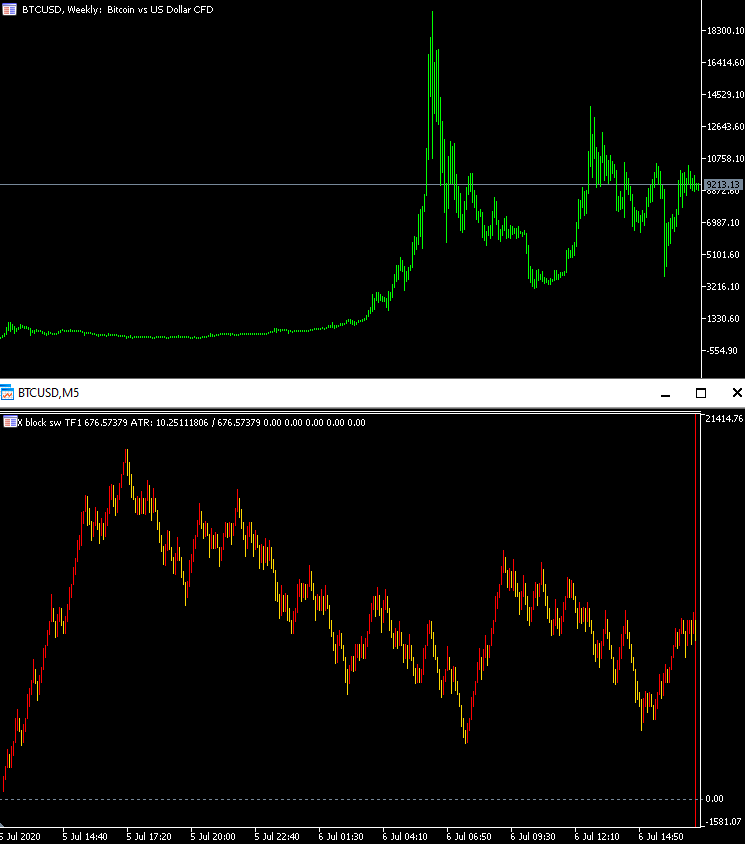Figure 10.

I came to the conclusion that the correct display is the one that takes into account the nature of the price series discreteness. However, since the nature of discreteness remains unknown, it will be "more correct" to use such a discretization method that can reflect the generation of profit/loss both by me and by other market participants. So, it is better to analyze the data on the basis of which our earnings are formed. That is, we should not consider the price movement in time, but we should only take into account by how much the price has moved.

An alternative to time discretization can be fixed-amplitude discretization, where it is assumed that the price is a function of itself. These are the blocks that we discussed earlier. A block of N points is considered to be closed after the price moves N points vertically. Perhaps this is not the best approach, but we are interested in exactly how many points the price has passed as this affects the profit or loss we generate. Moreover, the benefit of each participant changes just after the price has passed N points vertically. It means that the model in which the price is a function of itself is the closest to real trading. Figure 11 shows a sinusoid discretized in fixed-sized blocks. The shape is lost, but the main characteristics are preserved, including period and amplitude.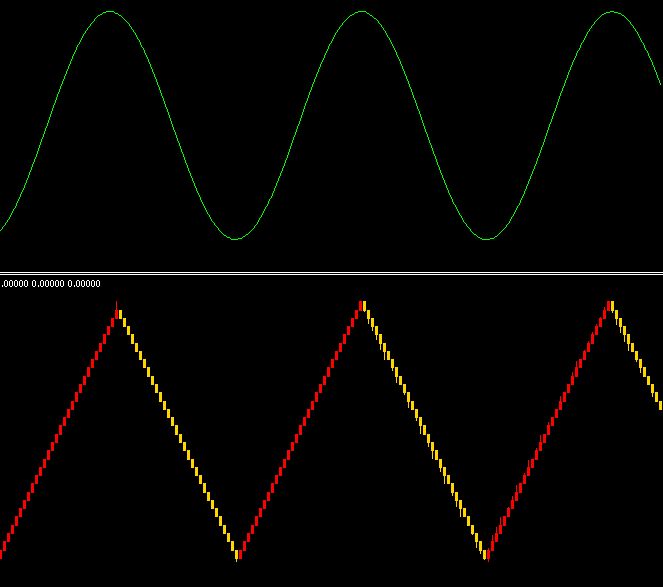Figure 11.

Even if incorrect discretization parameters are set, the values of the amplitude and period will still be preserved. Figure 12 shows an example of what happens when the block size is too large. Part of information which is inside the block is lost and thus the accuracy is worse (which is inevitable with data compression), but the information about the amplitude and the period is preserved. If the block size is larger than the price series amplitude, the blocks will not be displayed. So, in contrast to figure 2, if an incorrect discretization parameter is selected, the original deterministic signal does not turn into a random signal.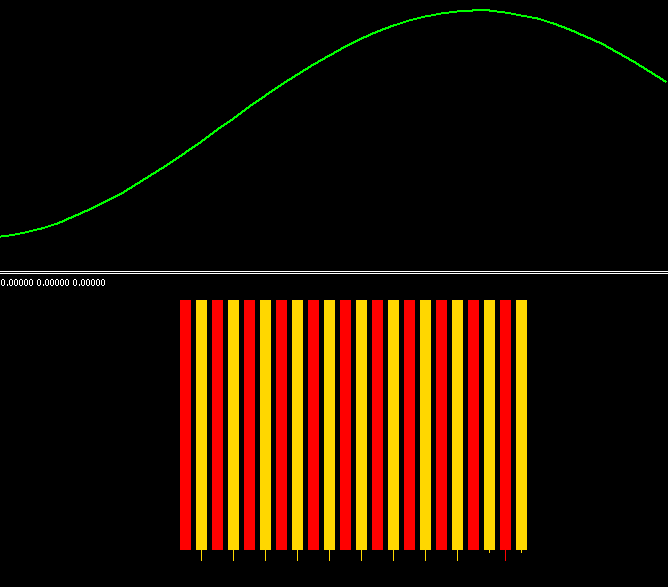Figure 12

This allows eliminating the random component introduced by a time discretized price series and in situations when it is unknown the function of which parameter the source series is. This method works better that time discretization and allows a convenient scaling of a chart, with a scaling change accuracy up to 1 point.

### Conclusion

• The nature of the price series is discrete, which stems from the pricing structure.
• A market price is not a function of time, but it is a function of closely related economic processes and currently it is not possible to take them all into account.
• Discretization of a price series into time intervals introduces a significant random component; this distorts the real shape of the price chart, adds noise and non-stationarity to this complex process with unknown parameters.
• It is necessary to take into account the function of which parameter the price is, when developing price series discretization methods.
• The non-stationarity of a price series is formed, among other reasons, due to incorrect discretization parameters.
• Time discretized price charts can be analyzed in an effort to find patterns, but it is necessary to take into account the above aspects, in order to better understand the nature of a particular found pattern.
• The idea is to develop other price series discretization methods which introduce much less distortion in the original data. One of such methods is described in this article.
• Trading algorithms and statistical market studies should be developed taking into account the specific features of the used price series discretization.

Translated from Russian by MetaQuotes Software Corp.
Original article: https://www.mql5.com/ru/articles/8136

Attached files |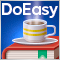Timeseries in DoEasy library (part 49): Multi-period multi-symbol multi-buffer standard indicators

In the current article, I will improve the library classes to implement the ability to develop multi-symbol multi-period standard indicators requiring several indicator buffers to display their data.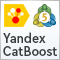Gradient Boosting (CatBoost) in the development of trading systems. A naive approach

Training the CatBoost classifier in Python and exporting the model to mql5, as well as parsing the model parameters and a custom strategy tester. The Python language and the MetaTrader 5 library are used for preparing the data and for training the model.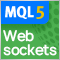Websockets for MetaTrader 5

Before the introduction of the network functionality provided with the updated MQL5 API, MetaTrader programs have been limited in their ability to connect and interface with websocket based services. But of course this has all changed, in this article we will explore the implementation of a websocket library in pure MQL5. A brief description of the websocket protocol will be given along with a step by step guide on how to use the resulting library.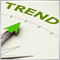What is a trend and is the market structure based on trend or flat?

Traders often talk about trends and flats but very few of them really understand what a trend/flat really is and even fewer are able to clearly explain these concepts. Discussing these basic terms is often beset by a solid set of prejudices and misconceptions. However, if we want to make profit, we need to understand the mathematical and logical meaning of these concepts. In this article, I will take a closer look at the essence of trend and flat, as well as try to define whether the market structure is based on trend, flat or something else. I will also consider the most optimal strategies for making profit on trend and flat markets.